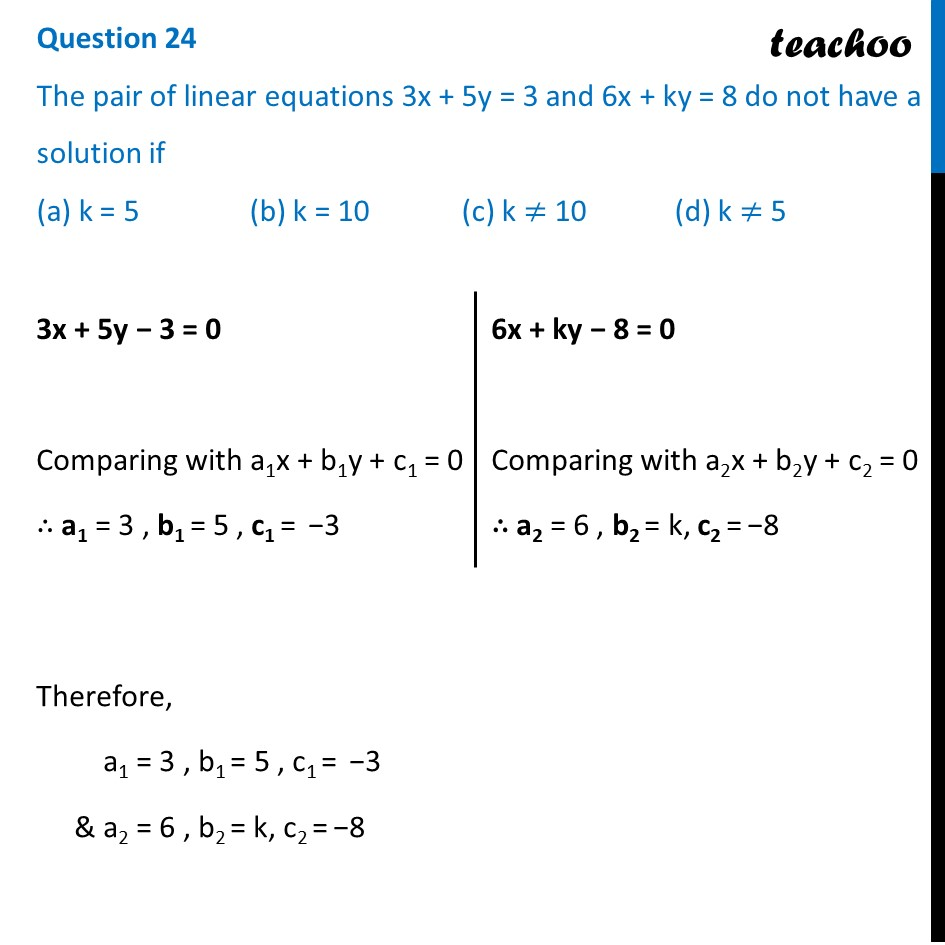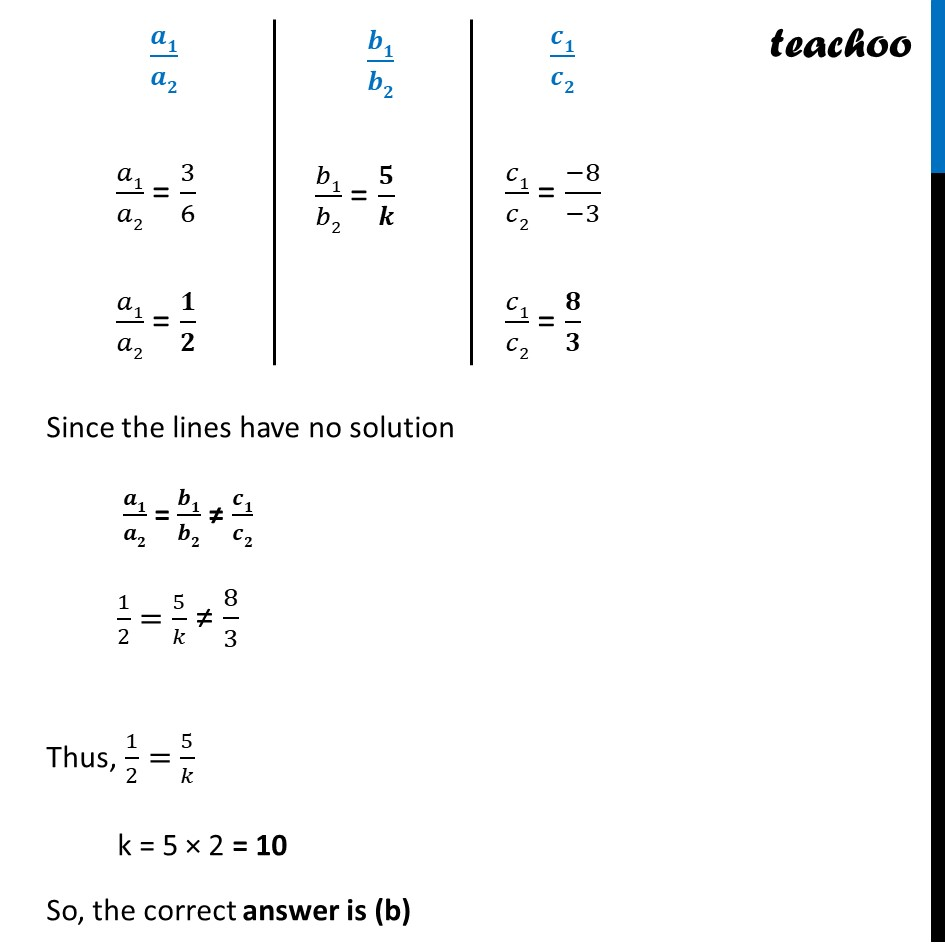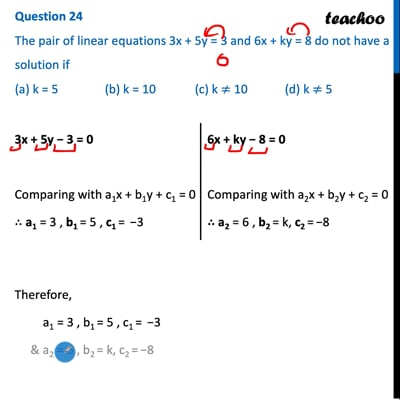CBSE Class 10 Sample Paper for 2022 Boards - Maths Basic [MCQ]

Class 10
Solutions of Sample Papers for Class 10 Boards

## (a) K = 5   (b) K = 10   (c) k ≠ 10   (d) k ≠ 5This video is only available for Teachoo black users

Solve all your doubts with Teachoo Black (new monthly pack available now!)

### Transcript

Question 24 The pair of linear equations 3x + 5y = 3 and 6x + ky = 8 do not have a solution if (a) K = 5 (b) K = 10 (c) k ≠ 10 (d) k ≠ 5 3x + 5y − 3 = 0 Comparing with a1x + b1y + c1 = 0 ∴ a1 = 3 , b1 = 5 , c1 = −3 6x + ky − 8 = 0 Comparing with a2x + b2y + c2 = 0 ∴ a2 = 6 , b2 = k, c2 = −8 Therefore, a1 = 3 , b1 = 5 , c1 = −3 & a2 = 6 , b2 = k, c2 = −8 𝑎1/𝑎2 = 3/6 𝑎1/𝑎2 = 𝟏/𝟐 𝑏1/𝑏2 = 𝟓/𝒌 𝑐1/𝑐2 = (−8)/(−3) 𝑐1/𝑐2 = 𝟖/𝟑 Since the lines have no solution 𝒂𝟏/𝒂𝟐 = 𝒃𝟏/𝒃𝟐 ≠ 𝒄𝟏/𝒄𝟐 1/2=5/𝑘 ≠ 8/3 Thus, 1/2=5/𝑘 k = 5 × 2 = 10 So, the correct answer is (b)